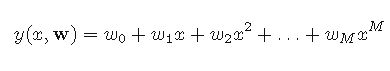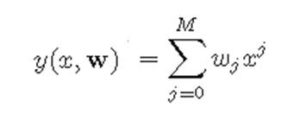# Machine Learning 101 – Polynomial Curve Fitting

This is Lecture 6 of Machine Learning 101. We would discuss Polynomial Curve Fitting.

Now don’t bother if the name makes it appear tough. This is simply a follow up of Lecture 5, where we discussed Regression Line.

So as before, we have a set of inputs

x = {x1, x2, . . . , xn}T where N = 6

corresponding to a set of target variables:

t = {t1, t2, . . . , tN}T where N = 6

Our objective is to find a function that relates each of the input variables to each of the target values.

If we assume that the relationship is a linear one, then we can use the equation of a straight line given as:

y = ß0 + ß1x

Then we simply calculates the coefficients  ß0 and  ß1

However since we don’t know the nature of this relationship, we would extend this equation to cover more options.

So we would have it as:which is the same as:This is similar to what we already have.  Just like y depends on x and ß in the linear model, also here, y depends on x and w.

M is the order of the polynomial. So if M is 1, then we have the linear model. If M is 2, then we have a quadratic function and so on.

So what is w?

w is simply the polynomial coefficients. So w0, w1, . . . , wM are denoted by the vector w.

So the problem reduces to simply determining the polynomial coefficients. Once we have it, we simple plug it into the polynomial and solve for x.

How do we determine w?

We determine w by fitting the polynomial to the training data set.  This is achieved by minimizing the error function that measures the difference between the function y(x,w), for any given value of w and the corresponding point in the training data set.

To perform minimization, we need the error function. A good choice is to use the sum of squares error between the predicted values y(xn, w)  for each training data point and the corresponding target values tn.

This error function is given by:where 1/2 is a factor added that would be explained later.

The value of this function would always be non-negative.  It can also be zero, but rarely. It is zero if and only if  the function produces exactly same output as the training set.

In summary, we need to choose the values of w that would produce the least value of E(w).

But another question is: How to  we choose M, that is the order of the polynomial?

I would explain this in the next lecture 6

Python Code for Polynomial Regression

```import numpy as np
import pandas as pd
import matplotlib.pyplot as plt
from sklearn.linear_model import LinearRegression
from sklearn.preprocessing import PolynomialFeatures

# Read the dataset into a dataframe

# Place the ranges in a variable and preprocess
x = df['x'].values
y = df['y'].values
x = x.reshape(-1, 1)

# Change the order here. degree is same as M
poly = PolynomialFeatures(degree=11)

# Fit a Polynomial Curve
X_poly = poly.fit_transform(x)
poly.fit(X_poly, y)
linreg = LinearRegression()
linreg.fit(X_poly, y)
y_pred = linreg.predict(X_poly)

# Plot the curves. The regression line is in red
plt.scatter(x,y, color='blue')
plt.plot(x, y_pred, color='red')
plt.show()
```#### kindsonthegenius

Kindson Munonye is currently completing his doctoral program in Software Engineering in Budapest University of Technology and Economics

View all posts by kindsonthegenius →